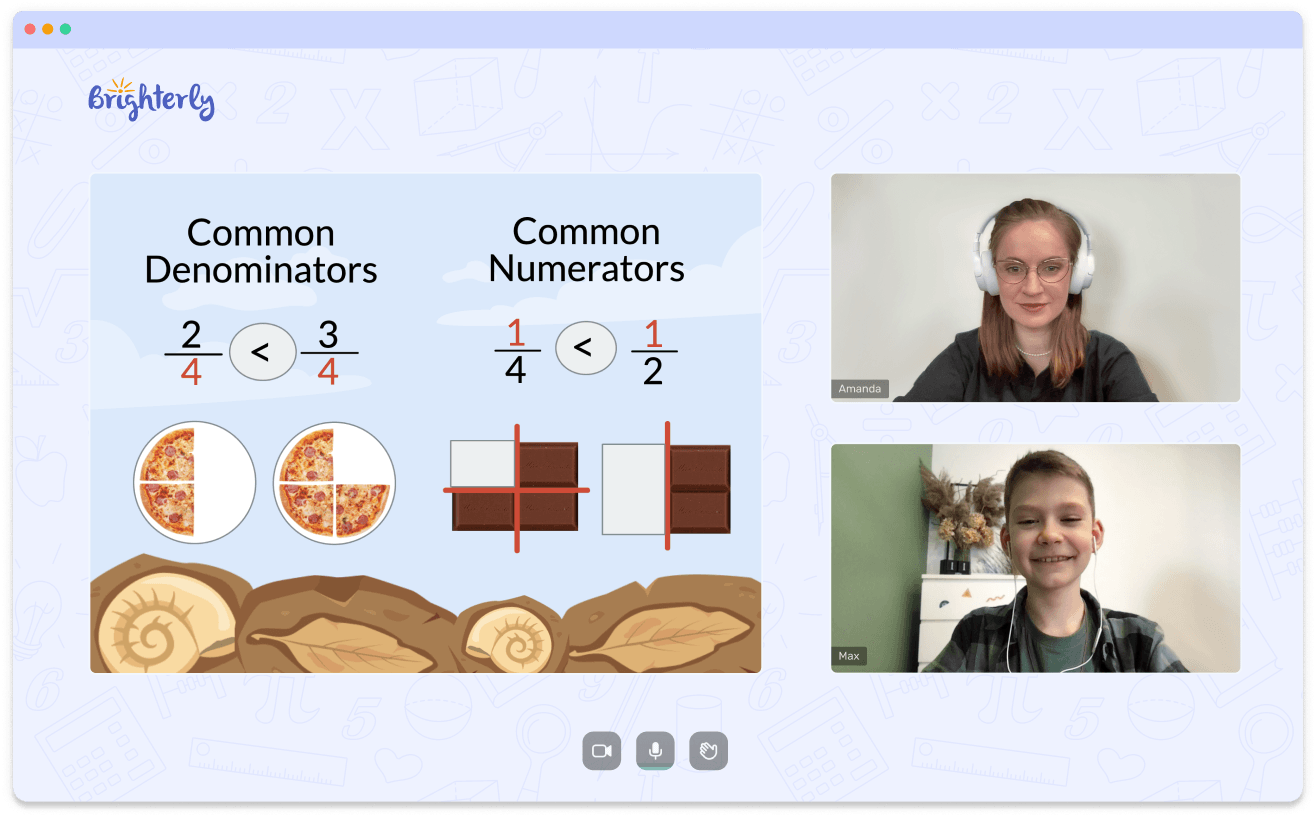# Multiplying Exponents Worksheets

Multiplying exponents worksheets help kids learn how to multiply large numbers faster. Using the multiplying exponents worksheet, a kid can learn about math facts, how to derive them, and how to remember them when needed. There are many benefits of using a multiplying exponents with same base worksheet.

## Benefits of multiplication of exponents worksheet

Here are some of the benefits of the multiplication of exponents worksheets:

Leave your email and we will send you worksheets

worksheets sent successfully

### Teachers can use them to set formative assessment tests for kids

As kids progress in a class, teachers often need to find out if they have understood the concept, and this goal can be achieved by setting assessment tests. Unfortunately, it is often a daunting task for teachers to set assessment tests from scratch, so they can use the multiplication property of exponents worksheets to set the tests instead.

Math for Kids

Is Your Child Struggling With Math?
1:1 Online Math Tutoring### Worksheets help kids remember the facts

When it comes to math facts, the easiest way a child can apply them in more complex situations is by remembering them. For example, kids can easily remember math facts with multiplication with exponents worksheets.

If you are a teacher looking to use the exponent multiplication worksheet as complementary teaching material, go online and check out math websites. You will find numerous types of multiplication of exponents worksheets to download and print for your kids.

Leave your email and we will send you worksheets

worksheets sent successfully### Multiplication of Exponents Worksheets PDF

Multiplication of Exponents Worksheets### Multiplication Property of Exponents Worksheets PDF

Multiplication Property of Exponents Worksheets### Multiplying Exponents with Same Base Worksheet PDF

Multiplying Exponents with Same Base Worksheet### Multiplying Exponents Worksheet PDF

Multiplying Exponents Worksheet

Book 1 to 1 Demo Lesson• We help children understand Math concepts instead of memorizing.
• We will adapt to your child needs to mprove math performance.

Is Your Child Struggling With Math?
Book 1 to 1 Demo Lesson